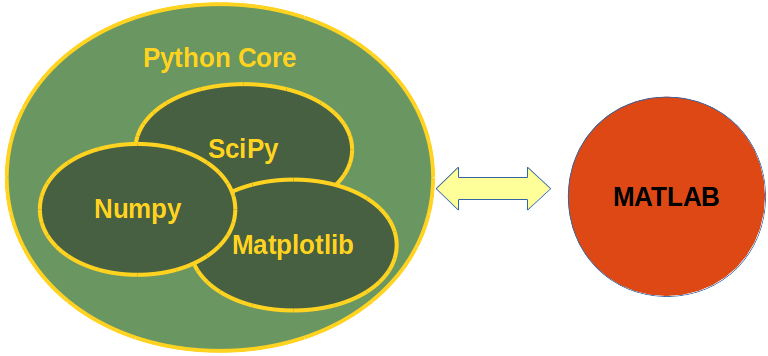## Tutorial and Online Course

### Data Science and Data AnalysisComment on our own account: Since October 2015 we are working on this tutorial on numerical programming in Python. Since then it has been the focus of our work. On the 10th of February 2016, we started translating the documentation into German..

This is a tutorial, which can be used as an online course on Data Science and Data Analysis. Data Analysis is widely understood as the science of managing and anlysing large datasets, or Big Data. It also means providing the tools to inspect, transform and model data. The goal mainly consists in gaining information.

### An Alternative to Matlab

Many scientists and engineers in the scientific and engineering world use R and MATLAB to solve their data analysis and data science problems. It's a question troubling lots of people, which language they should choose: The functionality of R was developed with statisticians in mind, whereas Python is a general-purpose language. Nevertheless, Python is also - in combination with its specialized modules, like Numpy, Scipy, Matplotlib, Pandas and so, - an ideal programming language for solving numerical problems. Furthermore, the community of Python is a lot larger and faster growing than the one from R. The principal disadvantage of MATLAB against Python are the costs. Python is completely free, whereas MATLAB can be very expensive. Python is a very attractive alternative of MATLAB: Python is not only free of costs, but its code is open source. Python is continually becoming more powerful by a rapidly growing number of specialized modules.

Python in combination with Numpy, Scipy and Matplotlib can be used as a replacement for MATLAB. The combination of NumPy, SciPy and Matplotlib is a free (meaning both "free" as in "free beer" and "free" as in "freedom") alternative to MATLAB. Even though MATLAB has a huge number of additional toolboxes available, NumPy has the advantage that Python is a more modern and complete programming language and - as we have said already before - is open source. SciPy adds even more MATLAB-like functionalities to Python. Python is rounded out in the direction of MATLAB with the module Matplotlib, which provides MATLAB-like plotting functionality.

### Data Anaysis